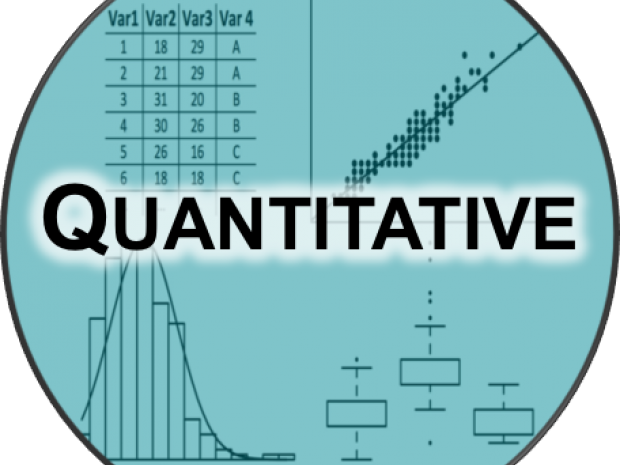Events

# Getting Started with MATLAB

Academic

For NYU CommunityMATLAB is a high-level technical computing language and interactive environment for algorithm development, data visualization, data analysis, and numerical computation. This introductory tutorial is designed to cover the basics of getting started with MATLAB as well as demonstrate some of the capabilities of MATLAB.

More information and to register: Getting Started with MATLAB